[Next] [Up] [Previous Section] [Next Section] [Home] [Other]

# Would a Perfect Computer be Old-Fashioned?

On most modern computers, the two most common sizes for floating-point numbers are 32 and 64 bits in length.

A 48-bit floating-point number can provide 10 decimal digits of precision. This is the same amount as provided by many electronic scientific calculators. It is fully adequate for many problems, and its appropriateness seems to have been established by long experience.

Thus, the NORC computer provided only one floating-point data type, which retained 13 significant digits of precision. As well, the Control Data 1604 and its successors such as the Control Data 3300 were popular computers used for advanced scientific work with a 48-bit word length; this word length was also used with many computers used for scientific computation in the Soviet Union and the People's Republic of China.

And a paper by Werner Bucholz on the design of the IBM 701 computer noted that its 36-bit word was chosen on the basis that a numeric precision below 10 digits would mean that double precision would be required by too many problems, while one above 12 digits would be needed for too few problems to be worthwhile.

If one is using 36-bit and 48-bit floating-point types, then, for double-precision, one might note that a 64-bit double-precision number almost always has more precision than is needed, and so one might follow the example of the Control Data 6600, which used 60 bits for floating point numbers, which were generally accepted to be adequate, as far as I know, by everyone concerned.

After some thought on the matter, I decided that the following floating-point formats, based on those of the IEEE 754 standard, but with modifications, would be appropriate: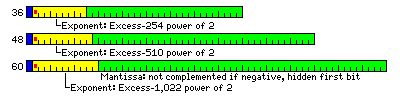These formats have the ranges:

```36 bit

with full precision

-77               77
6.9089 * 10    to 1.1579 * 10

-253                 256
( 0.5 * 2     to 0.9999... * 2    )

with reduced precision, down to

-85
5.1476 * 2

-279
( 0.5 * 2     )

48 bit

with full precision

-154               154
2.9833 * 10     to 1.3408 * 10

-509                 512
( 0.5 * 2     to 0.9999... * 2    )

with reduced precision, down to

48 bit

-166
1.3567 * 10

-550
( 0.5 * 2     )

60 bit

with full precision

-308               308
2.2164 * 10     to 1.7977 * 10

-1,021                 1,024
( 0.5 * 2       to 0.9999... * 2      )

with reduced precision, down to

-322
1.5810 * 10

-1,069
( 0.5 * 2       )
```

The precisions provided by these three formats are:

• 36 bits: 26 bits of mantissa plus one hidden first bit; 8.13 digits
• 48 bits: 37 bits of mantissa plus one hidden first bit; 11.44 digits
• 60 bits: 48 bits of mantissa plus one hidden first bit; 14.75 digits

In the IEEE 754 standard, eight bits are allocated for the exponent and its sign in 32-bit single-precision numbers, and eleven bits are allocated for the exponent and its sign in 64-bit double-precision numbers.

Here, I am allocating nine bits for the exponent and its sign in 36-bit floating-point numbers, and ten bits for the exponent and its sign in 48-bit floating-point numbers.

Why am I proposing this? On the IBM 360, for example, single-precision and double-precision numbers both had the identical exponent range; having two different exponent ranges already creates a possibility for confusion, why worsen it by adding two additional possibilities?

The rationale behind increasing the size of the exponent by one bit for 36-bit floating-point numbers is this:

The exponent range for 32-bit floating-point numbers in the IEEE 754 standard is quite limited. It is made necessary by the need to maintain adequate precision while using a binary exponent (instead of, for example, a hexadeximal one) to maintain good numerical properties.

Once the precision is increased to 36 bits, because of the hidden first bit in the IEEE 754 format, it is possible to match the precision provided by the IBM 7090 computer while expanding the exponent field by one bit. This was the standard for a satisfactory single-precision floating-point precision that I was trying to equal.

And increasing the length of the exponent field was highly desirable, as it extended the exponent range to approximately match that of the floating-point on the IBM 360. Thus, the result is a single-precision floating-point format that would equal or exceed the characteristics of the single-precision floating-point numbers on these two major platforms, as well as many others that resembled them.

In the case of 48-bit floating-point numbers, the rationale for making the exponent field one bit smaller than for double-precision is this:

The goal behind this format was to have a floating-point number format comparable to the numbers available from common electronic calculators. Such calculators typically displayed ten digits of precision, and had an exponent range from 10^99 to 10^-99.

The exponent range chosen is the minimum one necessary to extend as far as that. As well, by using only this exponent size, and not one more bit, allows the precision of the number to exceed 11 decimal digits. Since almost all electronic calculators that displayed ten digits of precision had at least one additional digit of precision internally, and many had two or three additional digits of precision, and in at least one case known to me, the Monroe 1655 programmable scientific calculator, an electronic calculator displayed ten digits, but performed calculations internally on 15-digit numbmers, it seemed important to have at least 11 decimal digits of precision if at all possible.

On the other hand, while significant savings in the time required for floating-point arithmetic could be achieved by reducing the precision if the straightforward techniques using a small number of gates are used, when advanced techniques like Wallace trees and carry-select adders are used, precision is a much less pressing concern. As well, random access memory is much less expensive than formerly. Thus, I am aware that what I am addressing in these pages is something of a non-problem at present.

If one divides memory into 12-bit cells, 36 bits uses three of them, 48 bits four, and 60 bits five. A 720-bit wide bus to memory would be excessive (but see this subsequent page).

### General Considerations

On the next page, the simplest way of accomodating 36-bit, 48-bit, and 60-bit floating-point formats will be considered; base the computer on a 12-bit word, and fetch three, four, or five of them for these types of floating-point numbers.

Later pages in this series, however, are going to examine ways of achieving a higher degree of efficiency in handling items of data of varying lengths.

On the IBM 360 computer, it was required to align 64-bit double-precision floating-point numbers on even eight-byte boundaries in memory, that is, at byte addresses that were an integer multiple of eight, as would be normal for a computer with a 64-bit word.

32-bit single-precision floating-point numbers, and 32-bit integers, could only be in the right or left half of such a word, though, not somewhere in the middle; they were on even four-byte boundaries in memory. This, of course, is what would be required for a computer with a 32-bit word.

Similarly, 16-bit halfword integers had to be on even two-byte boundaries. and nowhere else, as would be needed for a computer with a 16-bit word.

As it happened, the IBM 360 line of compatible machines included machines with 8, 16, 32 and 64 bit data buses to main memory. So these restrictions were precisely what was required to ensure that it would never be necessary to use two memory accesses, instead of one, to fetch a 32-bit number over a 32-bit data bus, or a 16-bit number over a 16-bit data bus, and so on.

This can be seen as a way to achieve the goal of alignment. If one is dealing with 36-bit, 48-bit, and 60-bit numbers, that same goal could be achieved if the data bus to memory was a rather large 720 bits wide. Attempting to approximate that condition will be examined on this page.

Achieving the goal of alignment allows maximum efficiency in retrieving items of the lengths with which we are concerned from memory.

However, there is a problem when this goal is achieved for a series of lengths not related by powers of two, the way those on the IBM 360 platform were.

If I wish to access the n-th element of an array of 36-bit numbers, stored contiguously, in a memory composed of 720-bit words, I have to divide n by twenty in order to calculate the address of the memory word I want. For 48-bit numbers, I have to divide by fifteen. For 60-bit numbers, I have to divide by twelve.

Excluding right shifts, I have to be able to divide by three and divide by five. Multiplying by those numbers can be performed by a simple shift and add. But there is no comparable way to speed up dividing by three and dividing by five through special-purpose circuitry to a level which would be reasonable as part of address calculation. This is the addressing problem.

A computer with twelve-bit words would solve that problem. So would a computer with a 48-bit word, but on which addresses pointed to individual six-bit characters.

But on such a computer, contiguous 36-bit and 60-bit numbers, while easy enough to point to, would keep crossing the boundaries of any natural size of memory word, whether 48 bits or 96 bits or 192 bits.

Some very tricky and ingenious schemes for meeting both the alignment condition and the addressing condition at the same time are going to be discussed on this page, and the page which follows it.

### Craziest of All

One other possibility, which I considered to be too extreme to be worth discussing, would be to use base-12 arithmetic for addressing. Then, division by three as well as division by four would be easy to perform on addresses, allowing the addressing condition and the alignment condition to be met simultaneously for items close to each other in length.

Decimal numbers can be represented by either bi-quinary notation or qui-binary notation, and, similarly, duodecimal numbers can be represented by bi-bi-ternary notation, bi-ter-binary notation, or ter-bi-binary notation:

```Decimal          Duodecimal
BQ    QB          BBT     BTB     TBB
5 1   2 1        6 3 1   6 2 1   4 2 1
----------       ----------------------
0   0 0   0 0    0   0 0 0   0 0 0   0 0 0
1   0 1   0 1    1   0 0 1   0 0 1   0 0 1
2   0 2   1 0    2   0 0 2   0 1 0   0 1 0
3   0 3   1 1    3   0 1 0   0 1 1   0 1 1
4   0 4   2 0    4   0 1 1   0 2 0   1 0 0
5   1 0   2 1    5   0 1 2   0 2 1   1 0 1
6   1 1   3 0    6   1 0 0   1 0 0   1 1 0
7   1 2   3 1    7   1 0 1   1 0 1   1 1 1
8   1 3   4 0    8   1 0 2   1 1 0   2 0 0
9   1 4   4 1    9   1 1 0   1 1 1   2 0 1
X   1 1 1   1 2 0   2 1 0
Y   1 1 2   1 2 1   2 1 1
```

Because this is equivalent to a mixed-radix notation, encoding in one system, and shifting over the component digits rather than a whole digit of the original base, and then decoding in another system allows divisions by factors of the base.

As three to the fifth power is 243, which is close to two to the eighth power, 256, what would be sought would be a form of Chen-Ho encoding which represented five digits at once, whether in base-6 or base-12.

One way to encode a five digit base-3 number in eight binary bits might proceed as follows:

Let the base-3 number be abcde. If neither bc and de are 22, then there are only eight possibilities for them, and so the eight bit number can be AAPPPQQQ where AA is 00, 01, or 10, representing the digit a, and PPP encodes the two digits bc, while QQQ encodes the two digits de.

Codes for the remaining possibilities begin with 11.

If only one of bc or de is 22, then we have the codes 11AA0PPP and 11AA1QQQ.

Then, the case where bcde is 2222, the code could be 1111AA00.

Five otherwise unencoded binary digits would be added to the code for base six, and ten otherwise unencoded binary digits would be added to the code for base twelve.

Thus, we have:

```abcde AAPPPQQQ
abc22 11AA0PPP
a22de 11AA1QQQ
a2222 1111AA00
```

as a diagrammatic summary of the coding scheme.

### An Initial Suggestion

One way that a computer could provide a 36-bit single precision floating-point word and a 48-bit intermediate precision floating-point word with a bus to memory that was 144 bits wide, and that would also provide for double-precision floating-point numbers that were 72 bits wide.

Since 72 bits is generous as regards precision, possibly the exponent field would be made wider for 72 bit floats. For 48-bit floats, initially I considered having two alternate forms of numbers at that length, allowing a choice between either of those two sizes for the exponent field. However, since I had specific goals in mind for what the 48-bit floating point format should achieve - to have an exponent range equivalent to a power-of-ten exponent of two decimal digits, plus sign, and a precision equivalent to ten decimal digits, ideally with as much extra precision as possible, so as to be comparable to what was found to be appropriate for scientific pocket calculators, as well as scientific desk calculators before them, such as the Wang 500 and 600 series, the Monroe 1655, and the HP 9100A, for example - it was possible to directly optimize the floating point format for those specific goals.Such a machine would have a 36-bit word as its basic unit, four of them being fetched at one time from memory.

In the previous section, a number of different formats were given for machine instructions composed of 16-bit halfwords. To have a base register indicate a 64 kilobyte span of memory, instead of a 4 kilobyte span of memory, the base register reference was moved in to the first word of the instruction. This was feasible, but it proved to result in a tight squeeze as far as opcode space was concerned. An 18-bit unit for instructions would allow a three-bit base register specification and a 15-bit address to share the same portion of an instruction, allowing orthogonal specification of memory to memory operations, and providing a generous supply of opcode space.

The basic memory-reference instructions on this architecture might look like this: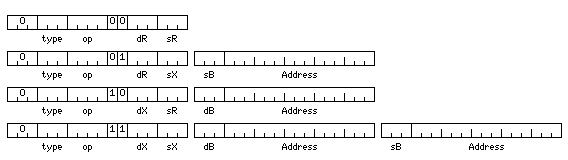Base register contents would be in units of a 144-bit memory extent; displacements might address an 18-bit unit in memory, as is required for a jump address; thus, there would be halfword arithmetic instructions, but individual characters that were 6, 8, 9 or even 16 bits long would only be accessed as part of string instructions. In the case of instructions acting on 48-bit floating-point values, the first 12 bits of the displacement would indicate which 144-bit memory extent was addressed, and the last 3 bits would contain only 0, 1, or 2, indicating which of the three aligned values in that extent was being accessed.

Another possibilty would be to simply abandon all pretense to originality whatsoever: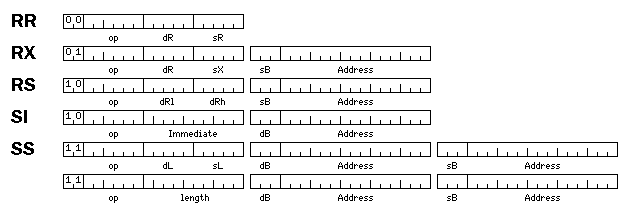General registers 24 through 31 would be the ones that could serve as base registers, thus allowing the displacements to be 15 bits long. Here, of course, addresses would be in units of a 9-bit character, following the practice in the architecture it slavishly imitates.

In addition to allowing the use of a 36-bit floating-point format with desirable properties, allowing each base register to refer to a larger area in memory, and, with a 36-bit word, perhaps not needing to move up to 72-bit addressing, it has the advantage of using sets of 32 registers, as are used on many RISC architectures.

One original feature might be included: when the base register field is zero, use post-indexed indirect addressing, which I have elsewhere called Array Mode, so that one can have several large arrays without having to allocate a base register to each one.

But there are other options. If indirect addressing is unacceptable, add the ability to handle more large arrays by having a 54-bit instruction format in which one memory address is simply included in the instruction as a 36-bit constant. As well, as I generally wish to include vector instructions similar to those of the Cray I and similar supercomputers, there are additional reasons to vary somewhat from the famous architecture I have used as a model here.

Another issue raised from attempting to slavishly transform the IBM System/360 architecture to a 36-bit word length is that if addresses point to 9-bit characters in memory, as might be expected from a strict analogy to the original model, a 9-bit character does not contain an even number of 4-bit BCD digits. So do the 5-bit length fields in packed decimal instructions specify the number of 36-bit words that a packed decimal number occupies? If so, these instructions are now dealing with much longer packed decimal numbers, with much coarser increments of length, than on the original 360, sufficiently as to call the usefulness of this type of packed decimal capability into question.

A 36-bit word does have the flexibility to handle data of different lengths.

Two words are 72 bits in length. That can contain nine 8-bit bytes. As well, one could have a sign, an exponent sign, 10 bits for three exponent digits in Chen-Ho encoding, and 60 bits for eighteen mantissa digits in Chen-Ho encoding, for decimal floating point.

Only four bits are wasted, as well, by putting a 32-bit word in a 36-bit word.

And four words, 144 bits in length, can be used to contain three 48-bit data items.

However, in some ways, the flexibility of a 36-bit word is limited; for example, a 576-bit wide bus to memory would be needed to hold nine 64-bit double-precision floating-point numbers, and a 1152-bit wide bus to memory would be needed to hold nineteen 60-bit double-precision floating-point numbers with 12 bits left over. Thus, unlike using 12, 24, and 48 bits as the basic unit, the flexibility is limited enough that it would likely be imperative to settle for using the natural 72-bit length for double-precision floating-point numbers.

As noted above, exactly following the System/360 might not be ideal. One possible set of basic instruction formats might be: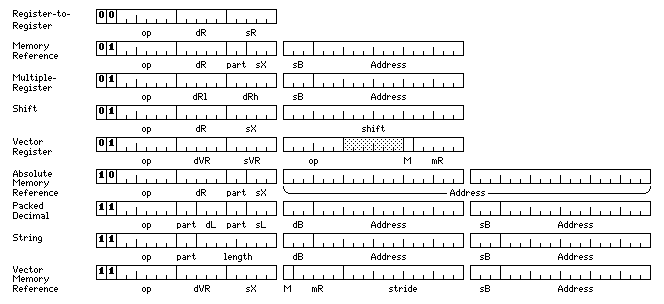Here, the 15-bit displacements are word displacements, with a two-bit part field in the first word of the instruction where appropriate. Thus, only general registers 1 through 7 are used as index registers.

In the case of packed decimal instructions, the part field would indicate one of three 12-bit portions of a 36-bit word, instead of one of four 9-bit characters in a 36-bit word, its usual function.

The vector register instructions, having additional available space, include their main opcode field in the second 18-bit halfword of the instruction, thus limiting the amount of opcode space they consume.

Thus, one-quarter of the opcode space is used for a complete set of register-to-register instructions that are 18 bits long; another quarter is used for a complete set of memory-reference instructions that are 36 bits long, with the limited number of other instructions of the same length, such as shift, vector register, and multiple-register instructions being squeezed in the same area; this leaves a quarter of the opcode space for a complete set of absolute-address memory-reference instructions, with a final quarter containing vector memory-reference instructions, with the packed decimal and string instructions squeezed in.

Another way to design an instruction set closely modeled on that of the IBM System/360, but based on the 36-bit word and the 9-bit byte, would be the following: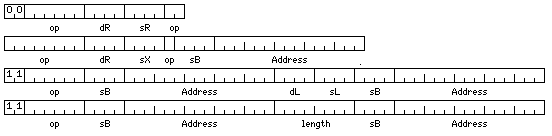Here, there are 16 general registers, all of which, except for register zero, are available for use as base and index registers, exactly as on the original System/360. The displacement field is still expanded to 15 bits from 12, and this does require some rearrangement of the fields within instructions.

It might be noted that the principle of having a 36-bit word, but fetching such words in groups of four, to allow additional factors of two in the number of bits in data objects handled, could be extended further.

For example, broader generality in the sizes of data types could be obtained with a 45-bit word length. If a machine were to fetch 16 such words from memory in a single operation (perhaps due to 4-way interleaving on a 180-bit wide bus), then the resulting 720-bit unit could be divided into:

• 90 8-bit characters
• 72 10-bit three-digit blocks
• 30 24-bit integers
• 20 36-bit single-precision floating-point numbers
• 15 48-bit intermediate-precision floating-point numbers
• 12 60-bit double-precision floating-point numbers

thus again providing the 36-bit single-precision floating-point number found so much more satisfactory on the IBM 7090 than the 32-bit single-precision that came later, but now no longer extending double-precision from 64 bits to 72 bits, but instead trimming it down to 60 bits, as was found perfectly adequate on the Control Data 6600 computer.

And, since 45 is divisible by 5, memory can also be divided into blocks of 10 bits, which can be used efficiently by means of Chen-Ho encoding or related systems to represent groups of three decimal digits!

Ironically, although such a scheme would allow handling of 8-bit and 16-bit wide quantities, as the only factors of 2 present are those from the number of words fetched, what this could not handle would be values that were 32 bits or 64 bits in size.

Of course, the reasons for favoring floating-point lengths of 36, 48, and 60 bits are somewhat vague: 36 bits because some programmers found the 7090 single precision adequate, but that of the System/360 inadequate (which led to DEC trying to squeeze everything out of the 32-bit floating-point on the PDP-11, ending up with the hidden first bit we still have today in the IEEE 754 standard), 48 bits because that can give 10 digits of precision, found on many pocket calculators, and 60 bits because that was good enough for the Control Data 6600.

The NORC computer suggests an alternative way of looking at floating-point precision. I have reason to believe its floating-point numbers had 13 digits of precision.

If so, this was certainly a brave choice! But if the computer is intended to calculate results for use in 10-figure tables, having three extra digits of precision carried throughout computations might be felt to be adequate in all but the most extraordinary cases.

I concluded that it had 13 digit precision from its exponent range, 10^31 to 10^(-43), or, for those with more modern browsers, 1031 to 10-43.

Let's suppose I want to be slightly more economical, but less brave. In a binary floating-point format, 10 bits of precision provide 3 digits of precision. So 40 bits provide 12 digits, and 43 bits would provide 12.944 digits of precision. Or 42 bits with a hidden leading 1 bit.

Some binary machines had a floating-point range of about 10^38 to 10^(-38), equivalent to 2^128 to 2^(-128), and others had one twice as large, from 10^76 to 10^(-76), equivalent to 2^256 to 2^(-256). Anything larger would mean that the exponent in a floating-point number would print with three digits, altering the expected format of numbers printed in exponential notation.

So this means eight bits for the exponent. And, of course, one extra bit for the exponent sign, and one extra bit for the sign of the number.

This adds up to a 53-bit word if the leading 1 bit is not hidden. As this is a prime number, perhaps it can be understood why there had never been a plethora of architectures with a 53-bit word.

### Perfection Within the Current Context

One possibility not considered so far, however, is to remain with the standard 8, 16, 32, and 64-bit division of memory, but to tolerate a small amount of waste. If we do not require that a floating-point number of presumed optimum length fit exactly into memory organized in this way, one can divide 128 bits into three words of 42 bits with two bits left over, or one can divide 256 bits into five words of 51 bits with one bit left over.

The IBM System/360 Model 44 had a knob on the front which allowed one to set it for certain jobs so that double-precision arithmetic could either remain unmodified, or it could run faster by ignoring the last 8, 16, or 24 bits of the mantissa of a double-precision number, giving effectively alternate floating-point sizes of 64, 56, 48, or 40 bits with mantissas of 56, 48, 40, and 32 bits in length, or 14, 12, 10 and 8 hexadecimal digits in length, this last set of numbers being the ones used to label the settings of that switch.

Inspired by this, desiring to continue to use a 64-bit unit, convenient to fetch by any implementation within the currently popular layout of memory, and yet not seeking to permit any waste of memory if it can be avoided, yet another alternative remains available.

When a floating-point multiplication is performed, only the mantissas of the floating-point numbers involved are multiplied. The exponents are merely added, a comparatively trivial and fast operation. And, so, one could have an intermediate-precision floating-point format that occupies 64 bits of memory, that has the precision one might expect from a 48-bit floating-point format (or one slightly larger), and that employs the bits that the reduced precision no longer requires as part of the mantissa to extend the exponent.

So, if a conventional 64-bit floating-point floating-point number uses one bit for the sign, 11 bits for an excess-1,024 exponent (or, in the case of IEEE-754, 11 bits for what I would term an excess-1,022 exponent, although the standard, putting the implied radix point of the mantissa, which it terms the significand, after the hidden first bit, speaks of it as an excess-1,023 exponent), and the remaining 52 bits for the mantissa, one could imagine that an intermediate-precision floating-point number which also occupies 64 bits could use one bit for the sign, 23 bits for the exponent, and 40 bits for the mantissa, thereby providing 12 digits of precision.

Of course, this also envisages constructing another Wallace Tree multiplier for the 40-bit mantissas, so that their products could be calculated more quickly than the products of the 52-bit mantissas of conventional double-precision numbers, with a third one for the still shorter mantissas of single-precision numbers. Thus, this assumes there is plenty of chip real estate available, and that obtaining the maximum possible floating-point performance is an overriding consideration.

Under such a circumstance, incidentally, one might also feel the need, due to the nature of Goldschmidt division, to drop, but only for division, the condition imposed by the IEEE 754 standard that the simple arithmetic operations always yield the best possible answer, even under the condition that what is truncated looks like .49999... or .50001... considering the mantissa as if it were an integer.

### Another Use for 51 Bits

Noting that 256 bits is only one more than 255 bits, and 255 is 5 times 51, allows us to add three bits to 48-bit floating-point numbers for almost an extra digit of precision.

51 bits, however, has another virtue as a word size. It is one more than a multiple of ten. Thus, with Chen-Ho encoding, it can be used to store fifteen decimal digits - and a sign.

Since 256 bits stores five such words, two memory words store ten of them, inviting the idea that these words could be used not simply as an auxilliary data type, but as the principal data type for a computer with decimal addressing.

Encoding decimal addresses with Chen-Ho encoding, and using the resulting string of bits as an address in a conventional memory array with a binary address, is not a new idea.

An example of the use of this principle was described in 7070/7074 Compatibility Feature for IBM System/370 Models 165, 165 II, and 168, IBM document number GA22-6958-1.

However, arbitrarily encoding addresses can create the difficulty that interleaved memory will no longer provide the expected benefits, as consecutive memory locations will no longer cycle through the available memory banks in order.

This, however, can be dealt with. One obvious, but slow, way, would be to simply convert the last three digits of the decimal address to the ten binary bits with the same numerical value instead of using Chen-Ho encoding.

However, the efficient Chen-Ho encoding of 1000 combinations of three decimal digits into 1024 combinations of ten binary bits can be scaled down (essentially, by eliminating the least significant bit of each digit) to an essentially identical coding of 125 combinations of three digits from 0 to 4 to 128 combinations of seven binary bits.

Instead of dividing a decimal number by eight, to encode the quotient in this way, and use the remainder as the last three bits of the address, an efficient scheme requiring only a limited amount of circuitry is possible.

Decimal digits may be represented in a number of codings; the conventional BCD or 8421 coding lends itself well to an immediate conversion into a quinary digit followed by a binary digit. The alternative 5421 encoding, on the other hand, is closely related to the bi-quinary representation of decimal digits.

```  8421 5421
0 0000 0000
1 0001 0001
2 0010 0010
3 0011 0011
4 0100 0100
5 0101 1000
6 0110 1001
7 0111 1010
8 1000 1011
9 1001 1100
```

Under 8421 encoding, the first three bits range from 000 to 100 only; under 5421 encoding, the last three bits range from 000 to 100 only.

Thus, using a small logic circuit to perform 5421 to 8421 translation, one can propagate the binary digits backwards and the quinary digits upward to create three quinary digits followed by three binary digits:

```8421 8421 8421
|||| |||| ||||
|||--|||--||||
||| |||| |||||
||| 5421 5421|
||| 8421 8421|
||| |||| |||||
||| |||--|||||
||| ||| ||||||
||| ||| 5421||
||| ||| 8421||
||| ||| ||||||
```

so, starting from the last three digits of an address in BCD format, one can get nine bits containing three quinary digits to efficiently encode to seven bits, followed by three bits containing the modulo-8 residue of the address.

This is pictured more fully below: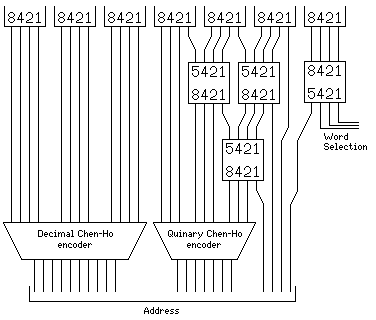Since ten 51 bit decimal words take up two 256-bit memory words, this would actually be applied to the three decimal digits immediately preceding, and exclusive of, the last decimal digit of the address of such a word. And, therefore, this addressing scheme would work well with up to 16-way interleaving. (Of course, with more layers of 5421 to 8421 conversion, even more binary digits could be propagated downwards if it were so desired.)

The treatment so far is, however, somewhat... conceptually unsophisticated.

A decimal Chen-Ho encoder can be formed by applying a quinary Chen-Ho encoder to the first three bits of each of three BCD digits and passing through the least significant bit of each BCD digit. Thus, there is no reason to stop propagating bits rightward exactly at the boundary of a group of three decimal digits.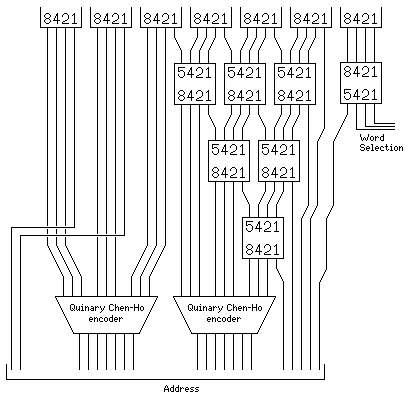Thus, if 32-way interleaving needed to be supported, the design could be extended as shown above.

### Back to the Model 44

Perhaps the simplest way to move to formats of these sizes for floating-point numbers would be to abandon any concern for conserving storage. Thus, one might go to a 36-bit word, and store 48-bit and 60-bit floating-point numbers in a 72-bit cell in memory, as illustrated below:This has the additional advantage of allowing compliance with FORTRAN standards, which state that INTEGER variables (and LOGICAL ones) should occupy the same space as a REAL variable, one "numeric storage unit", and DOUBLE PRECISION variables should occupy two numeric storage units.

I think that it is inappropriate for a language standard to make such specific demands on the underlying hardware; this could have been the reason why the Foxboro FOX-1 had a 24-bit floating-point format, unusably imprecise for most purposes in my view, and indeed it may have contributed to the rarity of 24-bit architectures, for which 48 bits is a reasonable single-precision floating point length, and 72 bits a reasonable double-precision floating point length.

The title of this section is a reference to the IBM System/360 Model 44, which had a knob on the front panel that could be used to reduce the precision of double-precision floating point numbers in units of 8 bits.

However, what I envisage is something more flexible. In addition to having instructions to operate on floating point numbers of each of the possible precisions, there would also be instructions to convert the numbers requiring 48 or 60 bits to packed form, so that they would only occupy 72 bits when part of a computation. Of course, that would usually be the case when they are in memory, so the conversion instructions would mainly be used in preparing for output, or converting after input.

Following the example of the Univac 1108, some memory-reference instructions could contain a four-bit "part" field; this would be sufficient to indicate these components of a 36-bit word:

```   1  The whole word
2  Two eighteen-bit halves
3  Three twelve-bit thirds
4  Four nine-bit characters
6  Six six-bit characters
----
16
```

This, however, suggests that it is time to note that this scheme is also applicable to other word lengths, particularly the 48-bit word, where the packed versions of the different floating-point formats, all being multiples of 12 bits in length, would pose no problems of addressing:Note that here, an additional complication is created by the fact that the 96-bit floats are not a longer version of double precision, but are instead a version of extended precision, in which the mantissa does not have a hidden first bit.

The alignment shown here seems to be the most natural way to simplify the floating ALU design.

However, the design built around the 36 bit word has the advantage that the feature of handling nonstandard precisions is easier to ignore; 72-bit double precision rather than 60-bit double precision can be used, and 48-bit intermediate precision need not be used. In the case of the 48-bit word, on the other hand, use of this feature is required in order to use single precision and double precision floats of sizes near to those most commonly used - 36 bits being near to 32 bits for single precision, and 60 bits being near to 64 bits for double precision.

[Next] [Up] [Previous Section] [Next Section] [Home] [Other]